# Force MotionPage 1

#### WATCH ALL SLIDES

Slide 1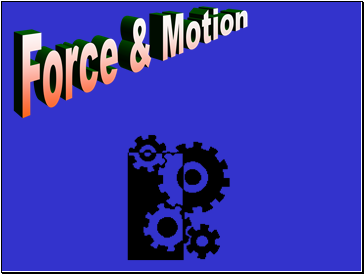Force & Motion

Slide 2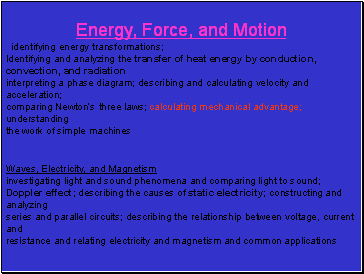## Energy, Force, and Motion

identifying energy transformations;

Identifying and analyzing the transfer of heat energy by conduction, convection, and radiation

interpreting a phase diagram; describing and calculating velocity and acceleration;

comparing Newton’s three laws; calculating mechanical advantage; understanding

the work of simple machines

Waves, Electricity, and Magnetism

investigating light and sound phenomena and comparing light to sound; Doppler effect; describing the causes of static electricity; constructing and analyzing

series and parallel circuits; describing the relationship between voltage, current and

resistance and relating electricity and magnetism and common applications

Slide 3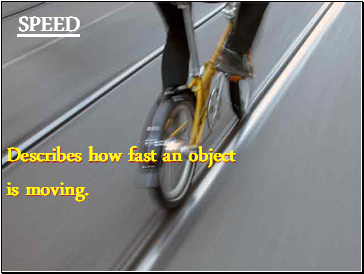SPEED

Describes how fast an object

is moving.

Slide 4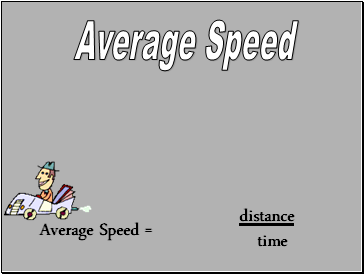## Average Speed

Average Speed =

distance

time

Slide 5## Velocity

The speed of an object in a certain direction.

Slide 6Velocity

V = velocity (meters/second)

d = distance (meters)

t = time (seconds)

d

V =

t

speed of an object in a certain direction.

Slide 7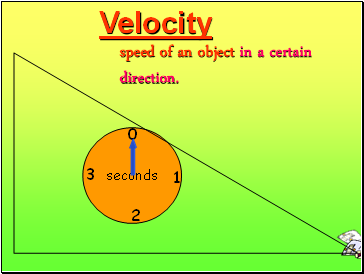Velocity

speed of an object in a certain direction.

seconds

0

2

1

3

Slide 8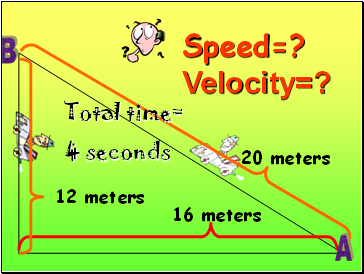Speed=?

Velocity=?

16 meters

12 meters

20 meters

Total time=

4 seconds

A

B

Slide 9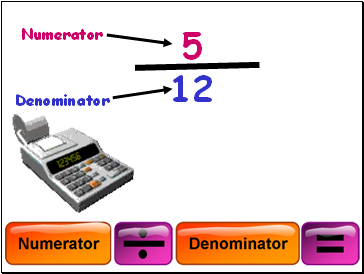Numerator

Denominator

5

12

Slide 10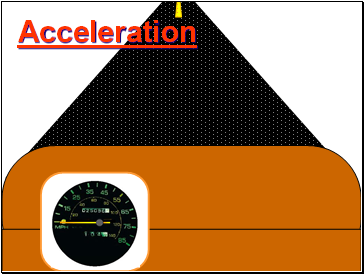## Acceleration

Slide 11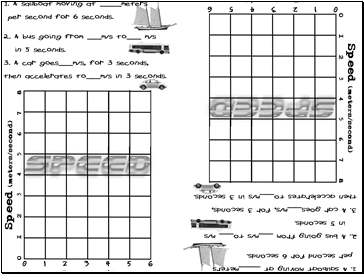Slide 12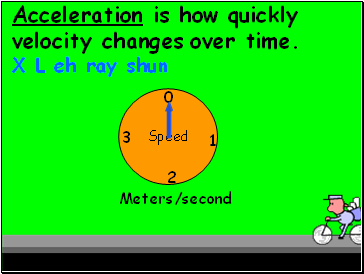Acceleration is how quickly velocity changes over time.

X L eh ray shun

Speed

0

2

1

3

Meters/second

Slide 13Acceleration

how quickly velocity changes over time.

A =

(Vfinal - Vinitial)

time

_

Slide 14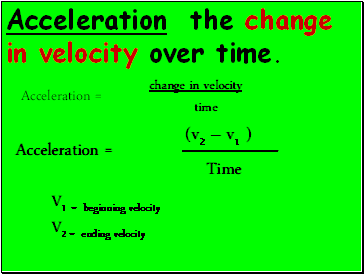Go to page:
1  2  3  4  5  6  7  8